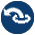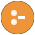#when.pl -- Conditional coroutining

This library implements the when/2 constraint, delaying a goal until its arguments are sufficiently instantiated. For example, the following delayes the execution of =:=/2 until the expression is instantiated.

```    ...
when(ground(Expr), 0 =:= Expr),```
author
- Tom Schrijvers (initial implementation)
- Jan Wielemaker
when(+Condition, :Goal)
Execute Goal when Condition is satisfied. I.e., Goal is executed as by call/1 if Condition is true when when/2 is called. Otherwise Goal is delayed until Condition becomes true. Condition is one of the following:
• `nonvar(X)`
• `ground(X)`
• ?=(X,Y)
• (Cond1,Cond2)
• (Cond2;Cond2)

For example (note the order `a` and `b` are written):

```?- when(nonvar(X), writeln(a)), writeln(b), X = x.
b
a
X = x```